NEET  >  Test: Work, Energy & Power

# Test: Work, Energy & Power

Test Description

## 25 Questions MCQ Test Topic-wise MCQ Tests for NEET | Test: Work, Energy & Power

Test: Work, Energy & Power for NEET 2022 is part of Topic-wise MCQ Tests for NEET preparation. The Test: Work, Energy & Power questions and answers have been prepared according to the NEET exam syllabus.The Test: Work, Energy & Power MCQs are made for NEET 2022 Exam. Find important definitions, questions, notes, meanings, examples, exercises, MCQs and online tests for Test: Work, Energy & Power below.
Solutions of Test: Work, Energy & Power questions in English are available as part of our Topic-wise MCQ Tests for NEET for NEET & Test: Work, Energy & Power solutions in Hindi for Topic-wise MCQ Tests for NEET course. Download more important topics, notes, lectures and mock test series for NEET Exam by signing up for free. Attempt Test: Work, Energy & Power | 25 questions in 50 minutes | Mock test for NEET preparation | Free important questions MCQ to study Topic-wise MCQ Tests for NEET for NEET Exam | Download free PDF with solutions
 1 Crore+ students have signed up on EduRev. Have you?
*Multiple options can be correct
Test: Work, Energy & Power - Question 1

### A person applies a constant force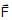on a particle of mass m and finds that the particle moves in a circle of radius r with a uniform speed v as seen (in the plane of motion) from an inertial frame of reference

Detailed Solution for Test: Work, Energy & Power - Question 1

The person applies ‘F’ constantly in a fixed direction. So, to keep the particle in constant circular motion of radius ‘r’ and uniform speed ‘v’, some other force whose resultant varies in magnitude and direction also act on the particle. Since the resultant of F and other force has to have constant magnitude but varying direction, the magnitude and direction of the other force has to change from point to point on circle.

*Multiple options can be correct
Test: Work, Energy & Power - Question 2

### A machine, in an amusement park, consists of a cage at the end of one arm, hinged at O. The cage revolves along a vertical circle of radius r (ABCDEFGH) about its hinge O, at constant linear speed v =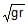. The cage is so attached that the man of weight `w' standing on a weighing machine, inside the cage, is always vertical. Then which of the following is correct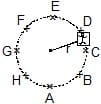Detailed Solution for Test: Work, Energy & Power - Question 2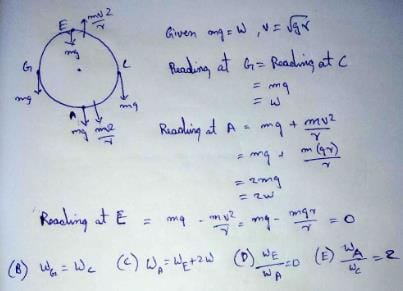*Multiple options can be correct
Test: Work, Energy & Power - Question 3

### A simple pendulum of length l and mass (bob) M is oscillating in a plane about a vertical line between angular limits _f and f. For an angular displacement q, [|q| < f] the tension in the string and velocity of the bob are T and v respectively. The following relations hold good under the above conditions :

Detailed Solution for Test: Work, Energy & Power - Question 3

The tangential acceleration is clearly gsinθ, by simply taking the components of the tension vector.
And the net for on the bob is
F = T – mgcosθ
And it will be equal to centripetal force, so,
T – mgcosθ = Mv2/l

Test: Work, Energy & Power - Question 4

A spot light S rotates in a horizontal plane with a constant angular velocity of 0.1 rad/s. The spot of light p moves along the wall at a distance 3 m. What is the velocity of the spot P when q = 45° ?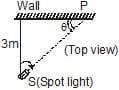Detailed Solution for Test: Work, Energy & Power - Question 4

Linear Velocity of rotating object ν=ωr/sinθ
r is the distance of object from centre.
Angle = 45o
r = d/cosθ [where d is the distance from the spot light to wall] = 3/cos45o
Velocity = ν = ωr/sinθ
= [0.1x3/cos45degree]/sin45degree
= 0.1x3/sin45 degreecos45degree
= 0.3/[1√2 x 1 /2]
= 2x0.3
= 0.6m/s
The velocity of the spot P is 0.6 m/s

Test: Work, Energy & Power - Question 5

Two moving particle P and Q are 10 m apart at a certain instant. The velocity of P is 8m/s making an angle 30° with the line joining P and Q and that of Q is 6m/s making an angle 30° with PQ as shown in the figure. Then angular velocity of P with respect to Q is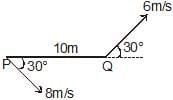Detailed Solution for Test: Work, Energy & Power - Question 5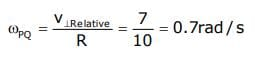Test: Work, Energy & Power - Question 6

The magnitude of displacement of a particle moving in a circle of radius a with constant angular speed w varies with time t as

Detailed Solution for Test: Work, Energy & Power - Question 6

If a particle is moving with angular velocity=ω
Its angle of rotation is given by ωt
Now displacement= length of line AB
Position vector of a particle is given by
R =iacosωt + jasinωt
Ro=ai
displacement =R−Ro
=a(cosωt−1)i+asinωj
d=√[(a(cosωt-1))2+(asinω)2]
=a√(2(1-cosωt))=a√(2×2(sinωt/2)2)=2asinωt/2

Test: Work, Energy & Power - Question 7

A particle moves along an arc of a circle of radius R. Its velocity depends on the distance covered as v = a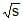, where a is a constant then the angle a between the vector of the total acceleration and the vector of velocity as a function of s will be

Detailed Solution for Test: Work, Energy & Power - Question 7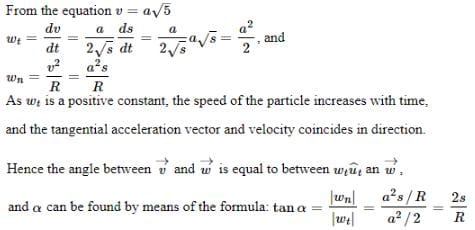Test: Work, Energy & Power - Question 8

A particle A moves along a circle of radius R = 50 cm so that its radius vector r relative to the point O (Fig.) rotates with the constant angular velocity w = 0.40 rad/s. Then modulus of the velocity of the particle, and the modulus of its total acceleration will be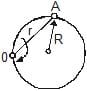Test: Work, Energy & Power - Question 9

Tangential acceleration of a particle moving in a circle of radius 1 m varies with time t as (initial velocity of particle is zero). Time after which total cceleration of particle makes and angle of 30º with radial acceleration is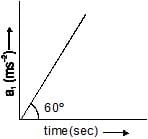Test: Work, Energy & Power - Question 10

A stone of mass of 16 kg is attached to a string 144 m long and is whirled in a horizontal smooth surface. The maximum tension the string can withstand is 16 newton. The maximum speed of revolution of the stone without breaking it, will be :

Detailed Solution for Test: Work, Energy & Power - Question 10

T= (mv2max)/r
16 = (16 v2max)/144
v2max = 12 m/s

*Multiple options can be correct
Test: Work, Energy & Power - Question 11

No work is done by a force on an object if

Detailed Solution for Test: Work, Energy & Power - Question 11

Work done = f.ds
So on perpendicular application for product becomes zero so no work is done

*Multiple options can be correct
Test: Work, Energy & Power - Question 12

One end of a light spring of spring constant k is fixed to a wall and the other end is tied to a block placed on a smooth horizontal surface. In a displacement, the work done by the spring is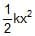. The possible cases are:

Detailed Solution for Test: Work, Energy & Power - Question 12

Stored elastic potential energy of spring =1/2​kx2 where x is compression or elongation of spring from its natural length. In this position the spring can do work on the block tied to it, which is equal to 1/2​kx2, so both option (a) & b are correct.

*Multiple options can be correct
Test: Work, Energy & Power - Question 13

Work done by force of friction

Detailed Solution for Test: Work, Energy & Power - Question 13

Since the motion of the body can be placed in the direction of friction, opposite to the direction of motion and even can not be placed in any motion.
So,  Work done by force of friction can be zero, negative, and can be positive.

*Multiple options can be correct
Test: Work, Energy & Power - Question 14

When total work done on a particle is positive

Detailed Solution for Test: Work, Energy & Power - Question 14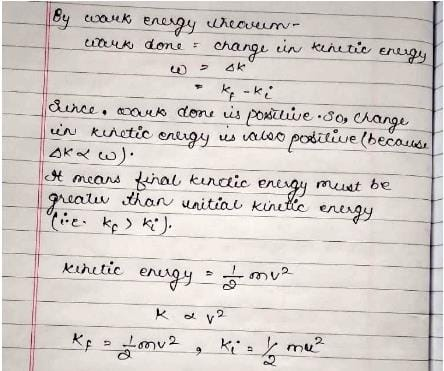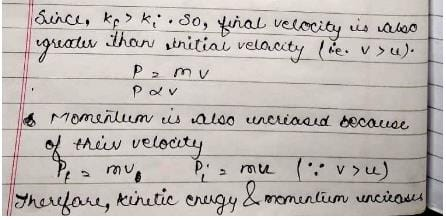*Multiple options can be correct
Test: Work, Energy & Power - Question 15

A particle with constant total energy E moves in one dimension in a region where the potential energy is U(x). The speed of the particle is zero where

Detailed Solution for Test: Work, Energy & Power - Question 15

If the total energy is E that means that E = U(x) + KE and if KE = 0 then E = U(x)

*Multiple options can be correct
Test: Work, Energy & Power - Question 16

A block of mass m slides down a plane inclined at an angle q. Which of the following will NOT increase the energy lost by the block due to friction ?

Detailed Solution for Test: Work, Energy & Power - Question 16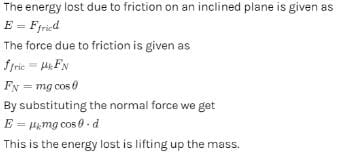Test: Work, Energy & Power - Question 17

A box of mass m is released from rest at position on the frictionless curved track shown. It slides a distance d along the track in time t to reach position 2, dropping a vertical distance h. Let v and a be the instantaneous speed and instantaneous acceleration, respectively, of the box at position 2. Which of the following equations is valid for this situation?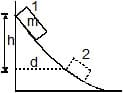Detailed Solution for Test: Work, Energy & Power - Question 17

According to the Work Energy Theorem,
Change in PE = Change in KE
So, mgh = ½ mv2
Hence C
Forum id- 1775160
For complete circle v = √(5gl)
mgh = ½mv2
So, h = 2.5R

*Multiple options can be correct
Test: Work, Energy & Power - Question 18

A ball of mass m is attached to the lower end of light vertical spring of force constant k. The upper end of the spring is fixed. The ball is released from rest with the spring at its normal (unstreched) length, comes to rest again after descending through a distance x.

Detailed Solution for Test: Work, Energy & Power - Question 18

The ball is at rest, it has maximum potential energy. When the ball is released from rest with the spring at its normal (unstretched) length it loses some potential energy and energy of spring increases. Hence, loss in potential energy of the ball is equal to gain in potential energy of spring.
∴mgx=1/2​kx2
∴x=2mg/k​
Also, for x′=x/2​,
kx′=mg i.e. forces are equal thus, the ball will have no acceleration at the position where it has descended through 2x​.
And when ball is at lowermost position, the spring force will be
kx=2mg
Hence, the ball will have an upward acceleration equal to g at its lowermost position.

*Multiple options can be correct
Test: Work, Energy & Power - Question 19

A cart moves with a constant speed along a horizontal circular path. From the cart, a particle is thrown up vertically with respect to the cart

Detailed Solution for Test: Work, Energy & Power - Question 19

The particle will land outside the circle because its initial velocity in horizontal direction is in the tangential direction and its point of projection is somewhere at the circumference of the circle.
Also its path will be parabolic because it is a case of projectile motion with velocity in horizontal as well as vertical direction.

*Multiple options can be correct
Test: Work, Energy & Power - Question 20

The potential energy in joules of a particle of mass 1 kg moving in a plane is given by U = 3x + 4y, the position coordinates of the point being x and y, measured in meters. If the particle is initially at rest at (6, 4), then

Detailed Solution for Test: Work, Energy & Power - Question 20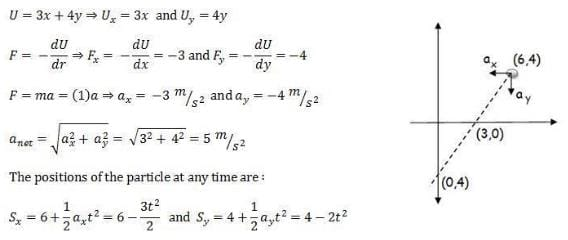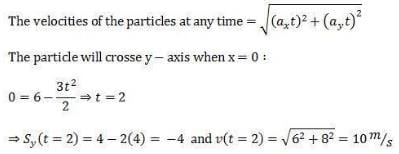Similarly you can find that the particle crosses x - axis at (3, 0) with a velocity 7.07 m/s

*Multiple options can be correct
Test: Work, Energy & Power - Question 21

A particle of mass m is released from a height H on a smooth curved surface which ends into a vertical loop of radius R, as shown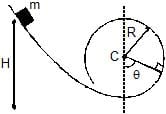Choose the correct alternative(s) if H = 2R

Detailed Solution for Test: Work, Energy & Power - Question 21

Velocity on reaching the base of circle is
u=√2gH=√2g2R=√4gR
This is less than √5gR. Hence, the particle cannot complete the circle. But this velocity is more than √2gR so the particle will break off the loop at a height above the centre before reaching the top.

*Multiple options can be correct
Test: Work, Energy & Power - Question 22

If q is instantaneous angle which the line joining the particle and the centre of the loop makes with the vertical, then identify the correct statement(s) related to the normal reaction N between the block and the surface

*Multiple options can be correct
Test: Work, Energy & Power - Question 23

The minimum value of H required so that the particle makes a complete vertical circle is given by

Detailed Solution for Test: Work, Energy & Power - Question 23

For complete circle v = √(5gl)
mgh = ½mv2
So, h = 2.5R

*Multiple options can be correct
Test: Work, Energy & Power - Question 24

Which of the following laws/principles of physics can be applied on the spring block system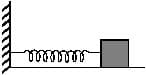Detailed Solution for Test: Work, Energy & Power - Question 24

Since friction is present in the scenario, so, only WET can be applied.

*Multiple options can be correct
Test: Work, Energy & Power - Question 25

The correct statement is

Detailed Solution for Test: Work, Energy & Power - Question 25

The question is incomplete and doesn’t make sense by itself. It should be changed to:
“A spring block system is placed on a rough horizontal floor. The block is pulled towards right to give spring an elongation less than 2μmg/K but more than μmg/K and released.
The correct statement is:”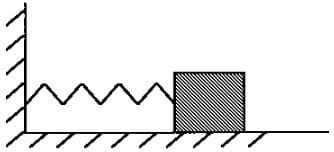Initially the spring block system will have only one form of energy and that will be spring potential energy stored in it. After the system is released from this position it starts to oscillate to and fro with friction acting on it. When the system comes to rest then the total stored potential energy will be converted into the work done by the frictional force on the block.

## Topic-wise MCQ Tests for NEET

1493 tests
 Use Code STAYHOME200 and get INR 200 additional OFF Use Coupon Code
Information about Test: Work, Energy & Power Page
In this test you can find the Exam questions for Test: Work, Energy & Power solved & explained in the simplest way possible. Besides giving Questions and answers for Test: Work, Energy & Power, EduRev gives you an ample number of Online tests for practice

## Topic-wise MCQ Tests for NEET

1493 tests

### How to Prepare for NEET

Read our guide to prepare for NEET which is created by Toppers & the best Teachers